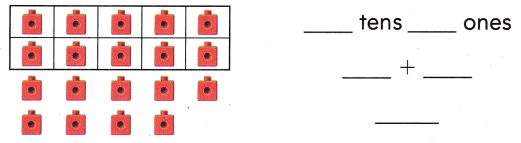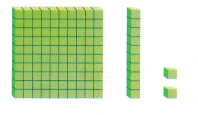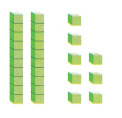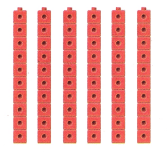Refer to our Texas Go Math Grade 1 Answer Key Pdf to score good marks in the exams. Test yourself by practicing the problems from Texas Go Math Grade 1 Module 1 Assessment Answer Key.

Vocabulary

Circle the ones in the number.
Draw a line under the tens in the number. (p.14)

Question 1.
48

Question 2.
84

Circle the hundred in the number.

Question 3.
108

Question 4.
119

Concepts and Skills

Use the model. Write the number three different ways. TEKS 1.2.A

Question 5.Write the numbers. TEKS 1.2.B

Question 6.__ hundred __ ten ___ ones = ___

Texas Test Prep

Question 7.
Which tells how many tens and ones? TEKS 1.2.B(A) 2 tens 8 ones
(B) 8 tens 2 ones
(C) 2 tens 9 ones

Question 8.
What number does the model show? TEKS 1.2.A(A) 50
(B) 60
(C) 6

Question 9.
What number is the same as 5 tens 6 ones? TEKS 1.2.B
(A) 55
(B) 65
(C) 56